## Turtle Solar SystemAre you ready to create your virtual own solar system? With a little Python code and a little math, the sky’s the limit!

Are you ready to write some code that’s out of this world? Using Python with Turtle, we’re going to create our own mini solar-system, with an earth that orbits the sun and a moon that orbits the earth.

### SETUP

Navigate to http://repl.it/languages/python_turtle (that’s “python_turtle”).

First, we need to import some stuff from library packages. Type this code into the left-hand editor:

```from turtle import Turtle, Screen
from math import sin, cos, pi, radians
```

Next, we need to set up a “Screen” object, which represents the empty void of space. We’re also going to create three “Turtle” objects, one for each celestial body. We could leave them as little triangles, but to make our solar system more elegant, let’s use the shape function to transform the sun, moon, and earth into circles. Finally, the penup function prevents our orbiting objects from scribbling all over our nice spatial void.

```screen = Screen()

sun = Turtle()
sun.shape('circle')

earth = Turtle()
earth.shape('circle')
earth.penup()

moon = Turtle()
moon.shape('circle')
moon.penup()
```

### THE EARTH ORBITS THE SUN

The sun sits at the centre of our solar system, and the planets spin around it in slow, whimsical ellipses. To simplify the math, we’re going to pretend the earth’s orbit is a perfect circle. So no matter what season it is — winter, spring, summer, or autumn — the earth is a fixed distance from the sun. Let’s add some variables to the code:

```earth_distance = 200
earth_angle = 0
```

Our planet starts at angle 0, and every day it inches almost 1° along its orbit, completing the full circle at 365 days / 360°. To make our code work, we need to figure out how to determine the exact position of the earth on any particular day.

### A LITTLE TRIGONOMETRY

Let’s put the earth on a cartesian graph. At the beginning, the earth starts 200 units to the right of the sun, otherwise known as the coordinates (200, 0). After circling around for 90°, our spunky little planet is directly above the sun at position (0, 200). Then, at 180° it reaches (-200, 0) and at 270° it hits (0, -200). But what about all the angles in between?

To figure that out, you start by transforming every point on the circle’s circumference into a right-angled triangle:

The radius of the circle becomes each triangle’s hypotenuse. And since we have right-angled triangles, we can use trigonometry!

At its heart, trig is about relationships between lengths. We know that the hypotenuse is always the longest side of a right-angled triangle. If the angle between the base and the hypotenuse is very big, then the opposite side is also very big. If that angle is very small, then the opposite side is very small. With trig, we can figure out exactly how big or small.

The sine function takes in the base angle and compares the opposite side with the hypotenuse. Let’s say our angle is 30°. sin(30) = 0.5, which means our opposite side is exactly 50% the length of the hypotenuse. If your hypotenuse is 8cm, then the opposite side is 4cm.

The cosine function does the same thing, but for the adjacent side. cos(30) = 0.866, so our adjacent side is roughly 87% the length of the hypotenuse (6.9com).

For any point on the circle’s circumference, the x and y coordinates are defined as follows:

x = hypotenuse length * sin(base angle)
y = hypotenuse length * cos(base angle)

Every time the angle increases by one degree, we get a new x and y position.

### THE ORBIT CODE

There are many different units we use to measure length. When you measure a piece of paper, you might use centimetres or inches. If you measure the distance from Los Angeles to Tokyo, you probably want kilometres or miles. Similarly, there are different units we use to measure angles. The most common is degrees, but there’s another system called radians, which is more suitable for trig. To keep things simple, we can use the radians function to automatically convert our degrees into the appropriate units.

All together, the code for our solar system goes like this:

```from turtle import Turtle, Screen
from math import sin, cos, pi, radians

screen = Screen()

sun = Turtle()
sun.shape('circle')

earth = Turtle()
earth.shape('circle')
earth.penup()

moon = Turtle()
moon.shape('circle')
moon.penup()

earth_distance = 200
earth_angle = 0

earth_x = earth_distance * cos(earth_angle)
earth_y = earth_distance * sin(earth_angle)
earth.setpos(earth_x, earth_y)

```

The setpos function places the earth at position (x, y), where the x and y coordinates are defined by our sin and cos functions. Every time we run the while loop, earth_angle is increased by one degree, until it completes the orbit. Hit the green “run” button at the top of the screen to try it out!

Adding our second celestial body is a little bit trickier. We know that the moon orbits the earth the same way the earth spins around the sun. But in this scenario, the earth isn’t standing still. Which means we need the moon to orbit the earth while orbiting the sun.

Let’s start by making the moon tag along at a fixed position, like a lovable but clingy puppy:

```while earth_angle <= radians(360):
earth_x = earth_distance * cos(earth_angle)
earth_y = earth_distance * sin(earth_angle)
earth.setpos(earth_x, earth_y)

moon.setpos(earth_x + 40, earth_y + 40)

```

Try it out!

To make the moon spin, we want to trade our fixed 40 unit offset with a variable offset that describes the moon’s orbit. And lucky for us, we already know how to define an orbit! We just need to create a new angle for the moon. Like the earth, the moon’s orbit increases at every iteration of the while loop.

It takes about 1 month for the moon to go through a full cycle. Since this is 12 times faster than the earth’s orbit, we’ll adjust our angles accordingly.

### FINAL CODE LISTING

```from turtle import Turtle, Screen
from math import sin, cos, pi, radians

screen = Screen()

sun = Turtle()
sun.shape('circle')

earth = Turtle()
earth.shape('circle')
earth.penup()

moon = Turtle()
moon.shape('circle')
moon.penup()

earth_distance = 200
earth_angle = 0
moon_distance = 40
moon_angle = 0

earth_x = earth_distance * cos(earth_angle)
earth_y = earth_distance * sin(earth_angle)
earth.setpos(earth_x, earth_y)

moon_x = moon_distance * cos(moon_angle)
moon_y = moon_distance * sin(moon_angle)
moon.setpos(earth_x + moon_x, earth_y + moon_y)

```

Psst! If you want to see something cool, delete the lines with the penup function and watch the celestial bodies trace their orbits!

#### Turtle programming-python

https://www.geeksforgeeks.org/turtle-programming-python/

#### Solar System

https://gist.github.com/CodeDotJS/64f0d3d86d05b93af3b6https://gist.github.com/CodeDotJS/64f0d3d86d05b93af3b6

#### Here comes the Whole solar system

http://firsttimeprogrammer.blogspot.com/2014/12/and-here-comes-whole-solar-system.html

#### Cartesian coordinates

https://www.mathsisfun.com/data/cartesian-coordinates.html

https://www.mathsisfun.com/data/graphs-index.html

#### Cartesian Plane

http://www.amathsdictionaryforkids.com/qr/c/CartesianPlane.html

#### Cartesian coordinate system

http://www.projectglobalawakening.com/cartesian-coordinate-system/

#### Pacman using Python

https://learn.wecode24.com/games-with-turtle-graphics/

## Author

•Patricia Foster is a computer science student at Carleton University. In addition to working professionally as a software developer, she spends her time reading and writing.

### Also In The October 2019 IssueBring out your virtual carving knives — it’s time to give your digital pumpkins some spooky faces!30+ ideas for STEAM-theme gifts for kids of all ages!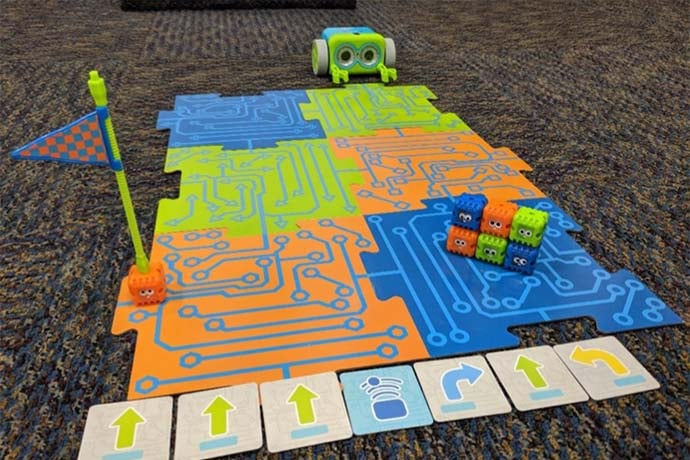Teach kids basic coding skills by letting them program Botley to zoom around the room, draw shapes, and even avoid obstacles!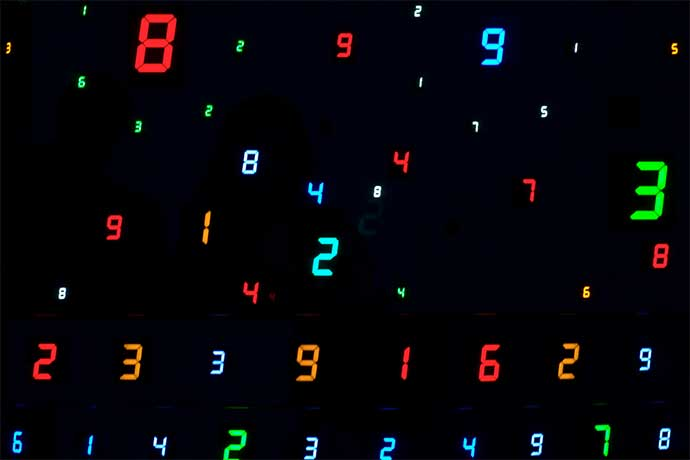Some numbers simply have a positive attitude. They're fun to play with.How 3D printing could help us get to Mars, and create new tools, homes, spacecrafts — even organs!No discussion of design is complete without the history of lorem ipsum. It's more than placeholder text you stuff into a visual design.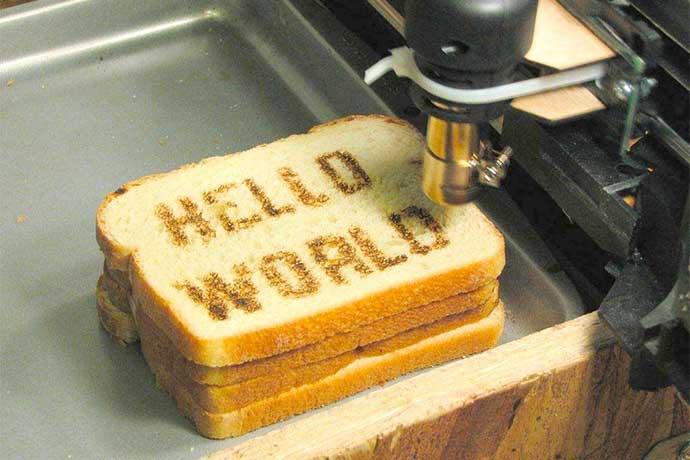"Hello World!" is one of the first programs you learn how to code. Here's the phrase in 4 languages with links to 100 more examples.Learn the delicious-sounding secrets that websites use to keep your passwords safe from hackers.A simple, quirky theorem with big applications, from picking socks to counting hairs.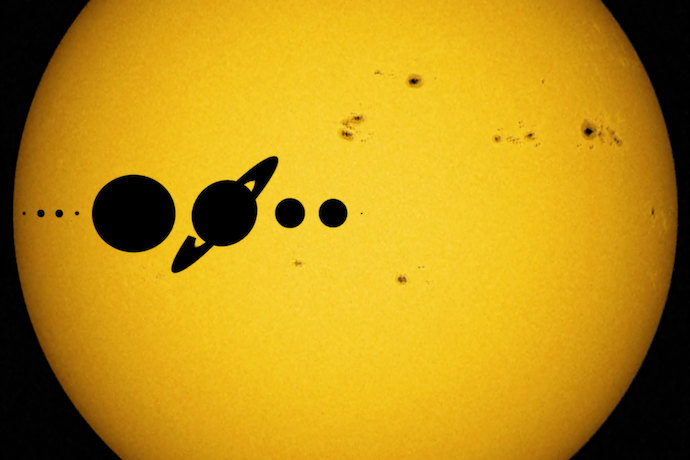Are you ready to create your virtual own solar system? With a little Python code and a little math, the sky’s the limit!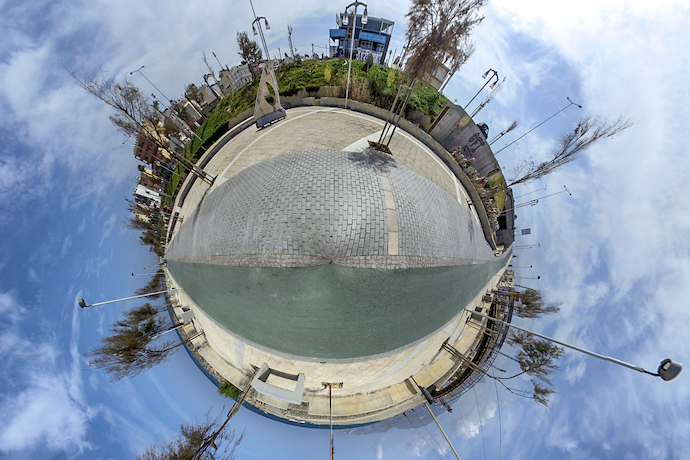Learn some of the tricks game developers use to simulate an extra dimension.There are pros and cons to networking all the “smart” devices in your home. What surprises does the future hold?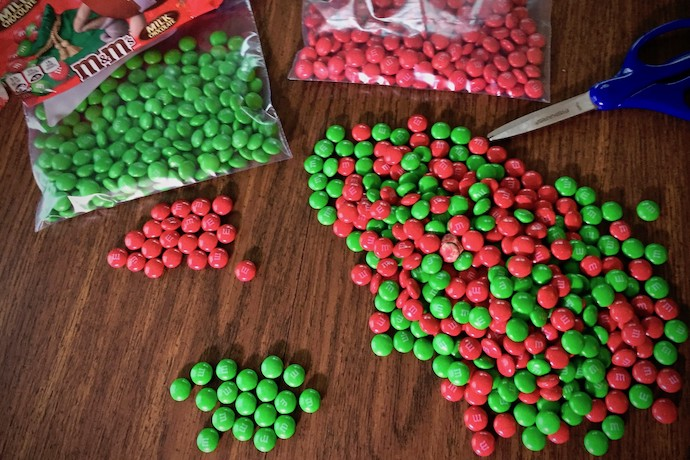Sometimes, even the most dynamic languages need to classify and check data. Now, you can add your own types to any language!Is it possible to steal software? And how do we know who owns code?Learn how to start making 3D games on this cool platform with over ninety million users.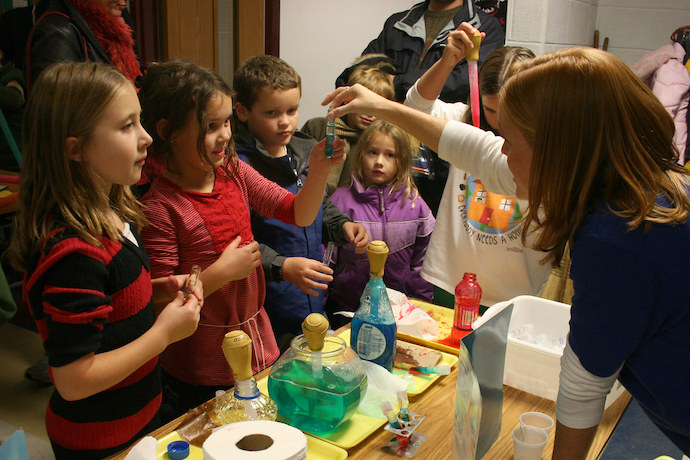Teaching strategies to better equip your students for a future full of open-ended problems.Check out this nifty feature that helps programs distinguish between variables with different scopes.Create a simple electronic game with CircuitPython and Adafruit, and test your reflexes against friends and family!Links from the bottom of all the October 2019 articles, collected in one place for you to print, share, or bookmark.Interested but not ready to subscribe? Sign-up for our free monthly email newsletter with curated site content and a new issue email announcement that we send every two months.

No, thanks!